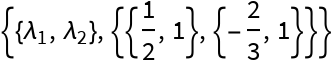Function Repository Resource:

AugmentedMatrix

Get the augmented matrix of the system of linear equations

Contributed by: Dennis M Schneider
 ResourceFunction["AugmentedMatrix"][eqns,vars] returns the augmented matrix of the system of equations eqns in the variables vars.

Details and Options

Matrix equations or a list of matrix equations are allowed.
If eqns is a list of expressions, then it is assumed that the right-hand sides are 0.

Examples

Basic Examples

Find the augmented matrix of a symbolic linear system:

 In:=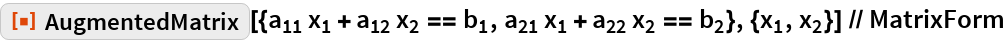Out=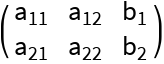Matrix equations are allowed:

 In:=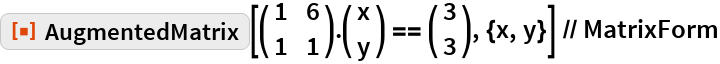Out=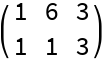When given a list of expressions, AugmentedMatrix assumes that the right-hand sides are 0:

 In:=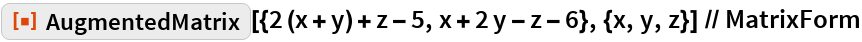Out=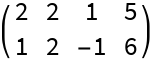A mixture of expressions and equations is allowed:

 In:=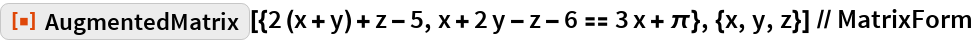Out=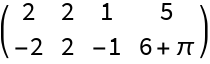Applications

Find all 2×2 matrices whose eigenvectors are (1,2) and (2,-3) :

 In:=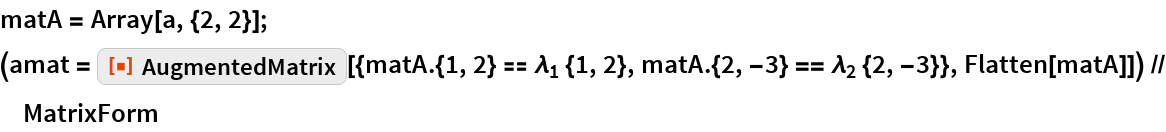Out=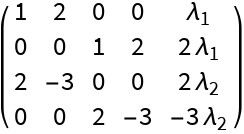Solve the system:

 In:=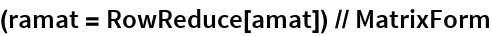Out=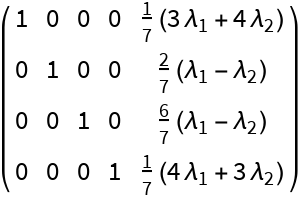The resulting matrix is:

 In:=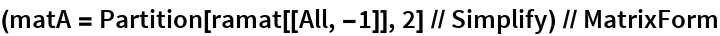Out=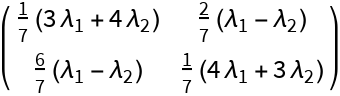Check that the result satisfies the appropriate eigensystem equation:

 In:=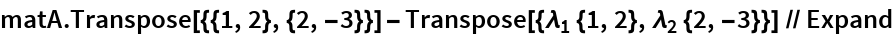Out=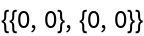Perform another check to ensure that the eigenvectors are as stated (up to scale):

 In:=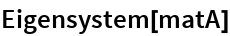Out=